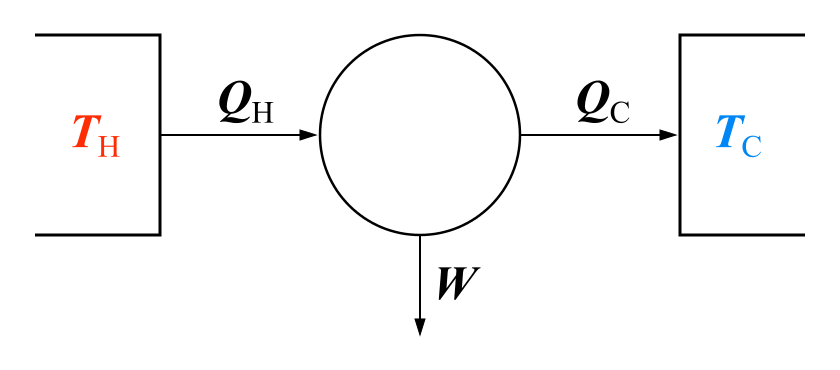# Difference Between First and Second Law of Thermodynamics

## Main Difference – First vs Second Law of Thermodynamics

Thermodynamics is a crucial part of physics, material sciences, engineering, chemistry, environment sciences and several other fields. There are four laws in thermodynamics; the zeroth law of thermodynamics, the first law of thermodynamics, the second law of thermodynamics and the third law of thermodynamics. These four laws assert that all the thermodynamic processes obey them. The first and second law are the most frequently used laws in thermodynamics. The first law says that energy can be neither created nor destroyed. The first law is simply another version of the law of conservation of energy. The second law, on the other hand, asserts that some thermodynamic processes are forbidden. This article focuses on the differences between the first and second law of thermodynamics.

## What is the First Law of Thermodynamics

The first law of thermodynamics is similar to the law of conservation of energy adjusted for thermodynamic processes. According to the law of conservation of energy, the total energy of an isolated system is constant. Energy cannot be created or destroyed but can be transformed from one form into another.

The first law states that the increase in internal energy of a closed system is equal to the heat supplied to the system minus work done by it. This statement can also be expressed as ΔU= ΔQ- ΔW where ΔU= the increase in internal energy, ΔQ= Heat supplied to the system,  and ΔW= Work done by the system. (ΔW is negative if work is done on the system.)

The first law sometimes expressed as ΔU= ΔQ+ ΔW. In this form of the first law, ΔW should be taken as the work done on the system. ΔW is negative if work is done by the system.

Anyhow, the first law asserts nothing about the ways of converting energy from one form to another.

## What is the Second Law of Thermodynamics

The second law of thermodynamics can be expressed in several ways as below.

It is impossible to build a perfect heat engine or a perfect refrigerator. This implies that a heat engine or a refrigerator with 100% energy efficiency cannot be constructed.

It is impossible to convert heat completely into work without some other change taking place. This statement says that energy is wasted whenever heat is converted into work. The amount of waste can be reduced. However, it cannot be eliminated.

It is impossible to build a perpetual motion machine. This statement implies that it is impossible to construct a perpetual motion machine as energy is wasted with time.

Heat can flow from a hot reservoir to a cold reservoir but not vice versa without some other change taking place. This statement implies that heat can be transferred from a hot reservoir to a cold reservoir without doing work. However, work must be done in order to transfer heat from a cold reservoir to a hot reservoir.

No heat engine can exist, having a thermal efficiency higher than that of a reversible Carnot engine. This statement implies that the thermal efficiency of a heat engine does not exceed the Carnot efficiency. The maximum possible thermal energy efficiency is called the Carnot efficiency. This concept is very helpful in science as it allows us to calculate the maximum achievable thermal efficiency of a given thermodynamic system.Working principle of Carnot’s heat engine

## Difference Between First and Second Law of Thermodynamics

### Basic idea:

First law:  First law of thermodynamics is a version of the law of conservation of energy.

Second law: Second law of thermodynamics states what types of thermodynamic processes are forbidden in nature.

### Content:

First law: First law of thermodynamics states that energy can be neither created nor destroyed.

Second law: It is impossible to construct a perfect heat engine or a perfect refrigerator. It is impossible to construct a perpetual motion machine. It is impossible to completely convert heat into work. Heat doesn’t spontaneously flow from a cold reservoir to a hot reservoir. The entropy of an isolated system never decreases.

### Uses:

First law: The equation; ΔU= ΔQ+ ΔW can be used to calculate the algebraic value of one quantity if other two quantities of the equation are known.

Second law: The second law can be used to calculate the maximum achievable thermal efficiency (Carnot efficiency) of a given heat engine.Image Courtesy:

“Carnot heat engine” by Eric Gaba (Sting – fr:Sting) – Own work Based upon Image:Carnot-engine.png, (Public Domain) via Commons Wikimedia